# Influence of system harmonics and negative sequence current on hydroelectric generator

School of Electrical and Electronic Engineering bookmark0 Lu Jiazheng (Hunan Electric Power Test Institute) bookmark1 Institute of Electrical and Electronic Engineering bookmark2 system harmonics and negative sequence current on the impact of hydroelectric generators operational safety hazards. The dq system model of salient-pole synchronous motor established by multi-loop method is used to derive the steady-state calculation method of stator rotor current, electromagnetic torque and damper strip current distribution when any harmonic or negative sequence is applied. Through the example calculation, the influence of power system harmonics and negative sequence on large hydro-generators is analyzed and illustrated.

1 The basic model of the salient-pole synchronous motor physical model and forward regulations. In order to calculate the distribution of the damper bar current, as shown, the d, q axis of the rotor is taken as the symmetry line, and the actual damping circuit is selected as the unit, and the voltage equation of each circuit is established, and the corresponding damper bar can be obtained after the loop current is obtained. Current.

In the analysis, the basic assumptions are: the generator is synchronous speed constant; the magnetic circuit saturation is not affected; each harmonic is a three-phase symmetrical sinusoidal waveform; according to the physical model of the salient pole synchronous motor and the selection method of the damping loop, Considering that each harmonic current voltage is three-phase symmetry, that is, there is no zero-axis component, so the voltage equation in the d, q, and 0 coordinate systems can be obtained as 1231 (m=n when each damper bar is even), which is an odd number. Steady-state current and torque calculation caused by m=n+1)2 harmonic voltage. The general form of any k-th harmonic (possibly positive sequence, possibly negative sequence) of sub-three-phase voltage is converted to d. After the q and 0 systems, the voltage and corresponding current of the jth harmonic in the d, q system can be expressed as substitution + and can be seen by equation (4). In general, if there is k harmonic voltage at the stator end, the stator In addition to the same k-order positive or negative sequence currents, the corresponding k*2 harmonic current will also occur due to rotor asymmetry.

22 The excitation winding current is obtained by the real part and the imaginary part of the excitation current caused by the kth harmonic voltage by the equation (2), then the corresponding actual excitation current can be obtained if k=1 and the terminal voltage is positive sequence, Ifu+1 ) = 0, if = If is the DC excitation current.

23 damper current calculation The real and imaginary parts of the d, q-axis damper loop current caused by the k-th harmonic voltage are solved by equation (2), then the d-axis ith damping loop current is 1t; q-axis ith is The damper loop current is based on the ith, / damper strip current caused by the kth harmonic voltage from the above loop currents (set m = n). If the number of dampers per pole is odd, then m = n + 1, then The current on the pole center line is i0=2imq. 24 The electromagnetic torque is obtained by superimposing the harmonic currents of the stator dq circuit and then superimposing to obtain the total current idiq, thereby obtaining the stator dq loop flux linkage, and the harmonics of each harmonic The total electromagnetic torque Tcm 3 example and the result analysis example, when it is connected in parallel in the power system, in addition to the rated fundamental wave, if there are high-order (5,7) harmonics at the same time, 5 harmonics The wave is negative sequence, and the 7th harmonic is positive sequence. It is assumed that the value of each harmonic voltage is 0.2. The corresponding stator current, field winding current and electromagnetic torque characteristics are as shown in the figure. The torque is the standard value. Since the air gap magnetic field generated by the two harmonic currents rotates relative to the rotor at 6 times synchronous speed, a current of 6 times frequency is induced in the rotor winding, and the harmonic magnetic field interacts with the excitation field of the rotor to generate 6 times frequency. Pulsating torque. Due to the asymmetry of the rotor, the 5th and 7th harmonic currents caused by the 5th and 7th harmonic voltages of the stator, the 6th harmonic current induced in the rotor will in turn cause the 7th and 5th harmonic components in the stator winding, respectively. But this component is relatively small. In addition, the actual value of the damper bar current corresponding to each harmonic is shown in Table 1. The actual value of the damper bar current corresponding to each harmonic of Table 1 (A) damper bar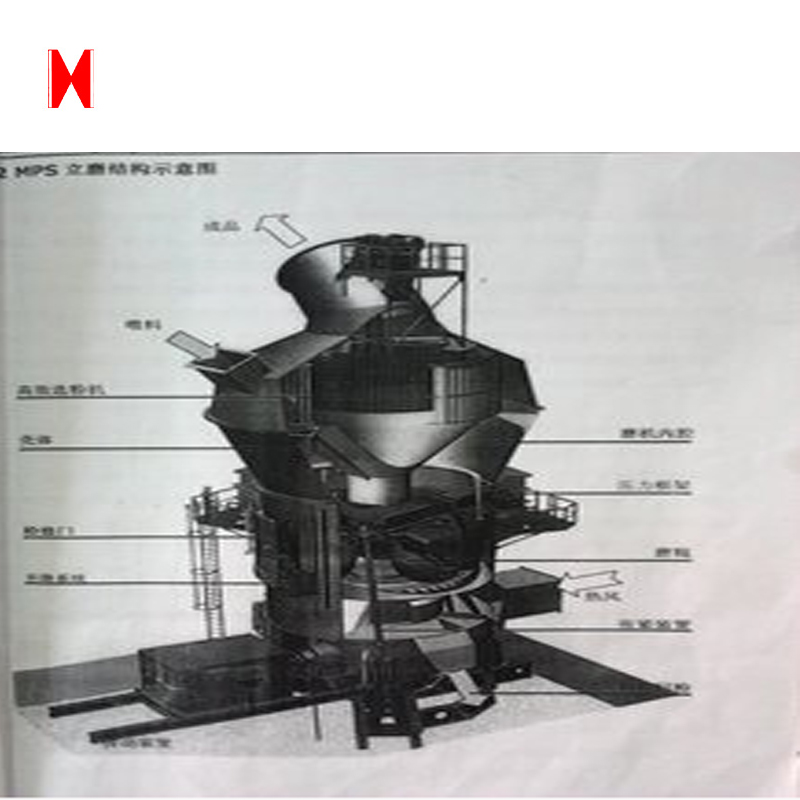Vertical Mill is an ideal large-scale grinding equipment, which is widely used in cement, electric power, metallurgy, chemical industry and non-gold mining industry. It has high production efficiency for broken, dry, pulverized, graded and transported, and it can be used to grind the bulk, granular and powdery materials into the required powdery materials.

The motor drives the mill through a reducer, The material falls from the feeding port to the center of the grinding disc. It moves to the edge of the grinding disc under the action of centrifugal force and is crushed by the grinding roller.The crushed material spills from the edge of the grinding disc, At the same time, it is carried by the hot air flow from the nozzle ring (wind ring) to the high efficiency separator with the vertical mill. The coarse powder is returned to the grinding disc after separation by separator. Fine powder is worn with the air flow and collected in the dust collecting device of the system, namely, the product. The coarse particles and unexpected metal parts that are not brought up by the hot gas flow sink down from the wind ring. After the scraper is scraped out, the extractor is fed into the mill and grinded again.

Company Introduction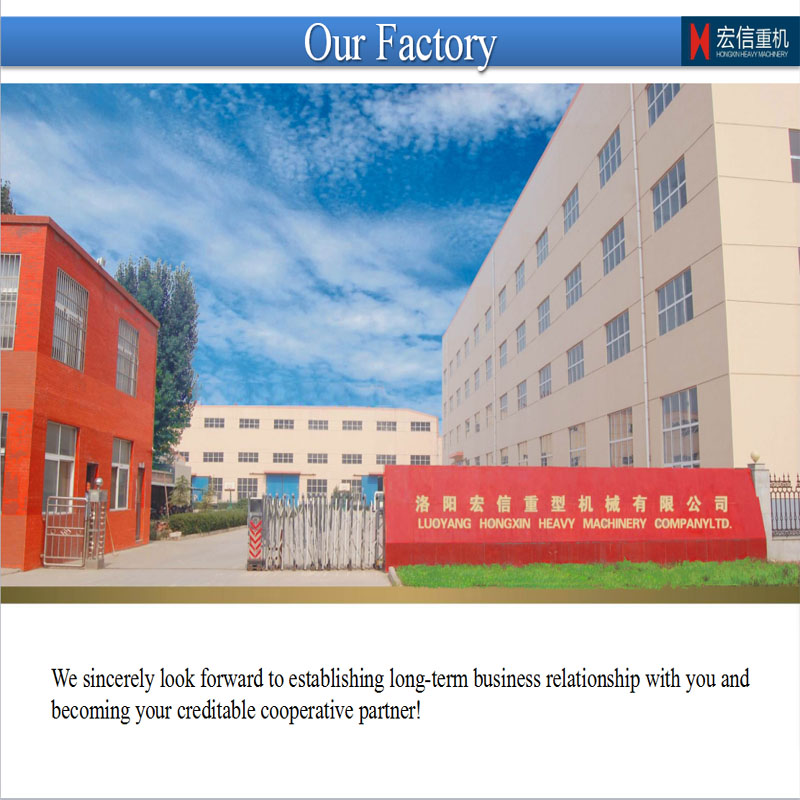Luoyang Hong Xin Heavy Co., LTD. is located in Luoyang Xin'an Industrial Park, Luoyang Hongxin Heavy Machinery Co., Ltd is an enterprise featured with industrial designing, processing and manufacturing, which is qualified for import-export trade. Covering an area of 15,000 square meters and holding an annual production value of 80 million RMB, the company of 20 million RMB registered capital has total 100 staff members, among which there are 20 engineering technicians owning senior and medium professional titles and 40 intermediate and senior technicians capable of product designing and developing.

Hongxin is dedicated to produce reducers, hoists, crushers, Ball Grinding Mills and the equipments of screening, coal washing, metallurgy and cement, and replacement parts. Meanwhile, we provide the perform maintenance service for various series of equipments. There are 52 large-scale equipments applied into the manufacturing process, such as T200 CNC floor boring and milling machine, YK 73125 CNC molding gear grinding machine, YK322B CNC molding gear grinding machine, Y3200 gear hobbing machine, Y1600 CNC gear sharper, 4m vertical lathe, 6.3m CNC vertical lathe, T110 CNC boring machine, and 52 middle-sized productive and assistive equipments, which integrate strong manufacturing and processing capability with complete managing system of production and technology, quality management system and comprehensive testing measures.

certificate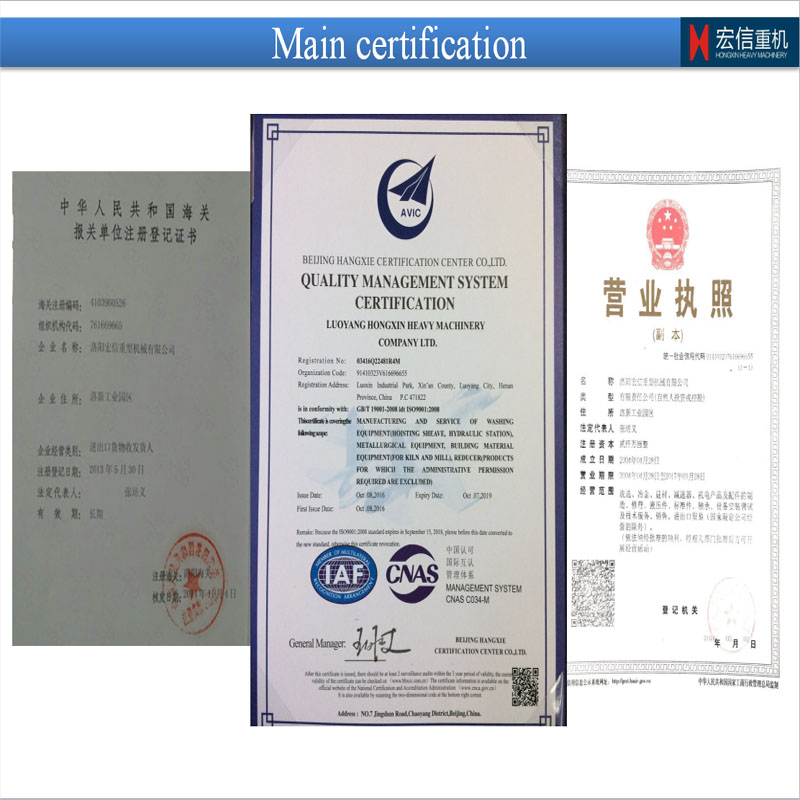contacts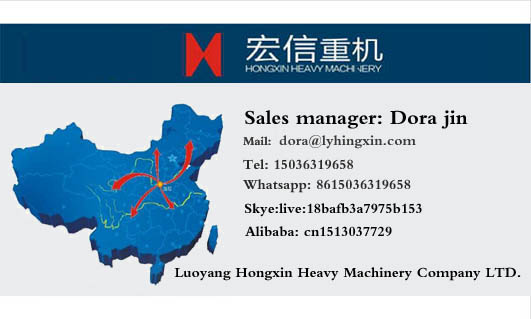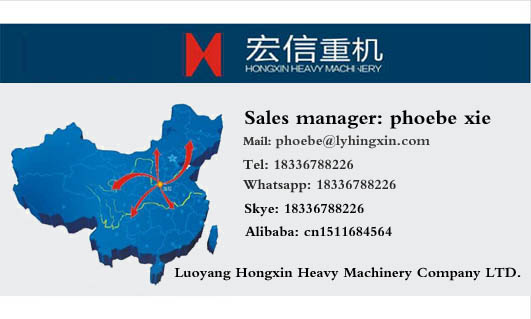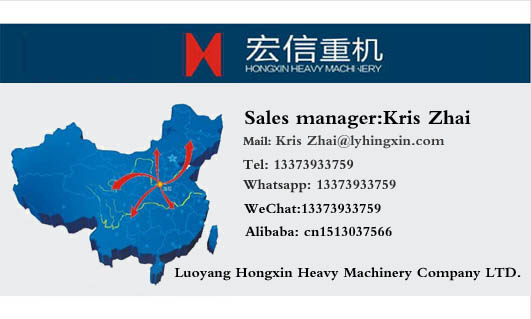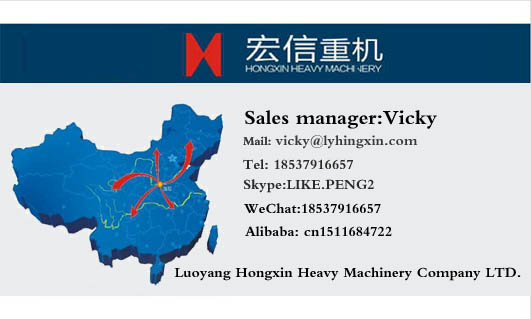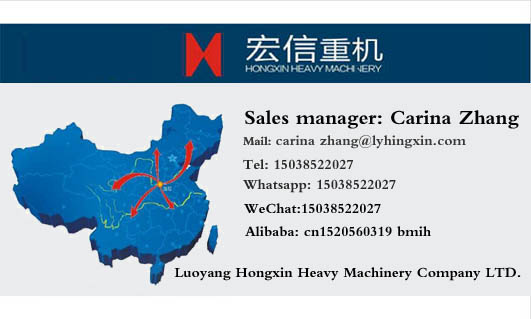Vertical Mill

Vertical Mill,Vertical Grinding Mill,Vertical Vibration Mill,Grinding Vertical Mill

Luoyang Hongxin Heavy Machinery CO., TLD. , https://www.hxreducer.com